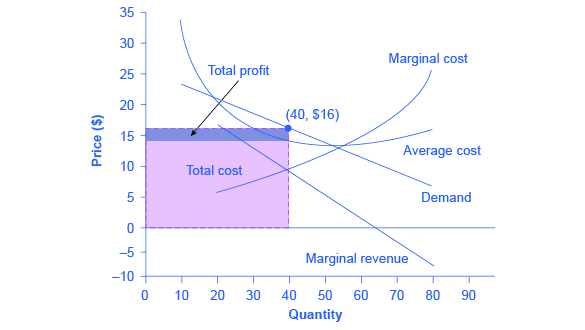## Choosing Price and Quantity

The monopolistically competitive firm decides on its profit-maximizing quantity and price in much the same way as a monopolist. A monopolistic competitor, like a monopolist, faces a downward-sloping demand curve, and so it will choose some combination of price and quantity along its perceived demand curve.

As an example of a profit-maximizing monopolistic competitor, consider the Authentic Chinese Pizza store, which serves pizza with cheese, sweet and sour sauce, and your choice of vegetables and meats. Although Authentic Chinese Pizza must compete against other pizza businesses and restaurants, it has a differentiated product. The firm’s perceived demand curve is downward sloping, as shown in Figure 10.3 and the first two columns of Table 10.1.Figure 10.3. How a Monopolistic Competitor Chooses its Profit Maximizing Output and Price To maximize profits, the Authentic Chinese Pizza shop would choose a quantity where marginal revenue equals marginal cost, or Q where MR = MC. Here it would choose a quantity of 40 and a price of $16. Table 10.1. Revenue and Cost Schedule Quantity Price Total Revenue Marginal Revenue Total Cost Marginal Cost Average Cost 10$23 $230$340 $34 20$20 $400$17 $400$6 $20 30$18 $540$14 $480$8 $16 40$16 $640$10 $580$10 $14.50 50$14 $700$6 $700$12 $14 60$12 $720$2 $840$14 $14 70$10 $700 –$2 $1,020$18 $14.57 80$8 $640 –$6 $1,280$26 $16 The combinations of price and quantity at each point on the demand curve can be multiplied to calculate the total revenue that the firm would receive, which is shown in the third column of Table 10.1. The fourth column, marginal revenue, is calculated as the change in total revenue divided by the change in quantity. The final columns of Table 10.1 show total cost, marginal cost, and average cost. As always, marginal cost is calculated by dividing the change in total cost by the change in quantity, while average cost is calculated by dividing total cost by quantity. The following example shows how these firms calculate how much of its product to supply at what price. ### How a Monopolistic Competitor Determines How Much to Produce and at What Price The process by which a monopolistic competitor chooses its profit-maximizing quantity and price resembles closely how a monopoly makes these decisions process. First, the firm selects the profit-maximizing quantity to produce. Then the firm decides what price to charge for that quantity. Step 1. The monopolistic competitor determines its profit-maximizing level of output. In this case, the Authentic Chinese Pizza company will determine the profit-maximizing quantity to produce by considering its marginal revenues and marginal costs. Two scenarios are possible: • If the firm is producing at a quantity of output where marginal revenue exceeds marginal cost, then the firm should keep expanding production, because each marginal unit is adding to profit by bringing in more revenue than its cost. In this way, the firm will produce up to the quantity where MR = MC. • If the firm is producing at a quantity where marginal costs exceed marginal revenue, then each marginal unit is costing more than the revenue it brings in, and the firm will increase its profits by reducing the quantity of output until MR = MC. In this example, MR and MC intersect at a quantity of 40, which is the profit-maximizing level of output for the firm. Step 2. The monopolistic competitor decides what price to charge. When the firm has determined its profit-maximizing quantity of output, it can then look to its perceived demand curve to find out what it can charge for that quantity of output. On the graph, this process can be shown as a vertical line reaching up through the profit-maximizing quantity until it hits the firm’s perceived demand curve. For Authentic Chinese Pizza, it should charge a price of$16 per pizza for a quantity of 40.

Once the firm has chosen price and quantity, it’s in a position to calculate total revenue, total cost, and profit. At a quantity of 40, the price of $16 lies above the average cost curve, so the firm is making economic profits. From Table 10.1 we can see that, at an output of 40, the firm’s total revenue is$640 and its total cost is $580, so profits are$60. In Figure 10.3, the firm’s total revenues are the rectangle with the quantity of 40 on the horizontal axis and the price of $16 on the vertical axis. The firm’s total costs are the light shaded rectangle with the same quantity of 40 on the horizontal axis but the average cost of$14.50 on the vertical axis. Profits are total revenues minus total costs, which is the shaded area above the average cost curve.

Although the process by which a monopolistic competitor makes decisions about quantity and price is similar to the way in which a monopolist makes such decisions, two differences are worth remembering. First, although both a monopolist and a monopolistic competitor face downward-sloping demand curves, the monopolist’s perceived demand curve is the market demand curve, while the perceived demand curve for a monopolistic competitor is based on the extent of its product differentiation and how many competitors it faces. Second, a monopolist is surrounded by barriers to entry and need not fear entry, but a monopolistic competitor who earns profits must expect the entry of firms with similar, but differentiated, products.

## Self Check: Calculating Cost, Revenue, Profit and Losses

Answer the question(s) below to see how well you understand the topics covered in the previous section. This short quiz does not count toward your grade in the class, and you can retake it an unlimited number of times.

You’ll have more success on the Self Check if you’ve completed the Reading in this section.

Use this quiz to check your understanding and decide whether to (1) study the previous section further or (2) move on to the next section.